Next: Specific Heat Up: Classical Thermodynamics Previous: Introduction

# Ideal Gas Equation of State

Let us commence our discussion of classical thermodynamics by considering the simplest possible macroscopic system, which is an ideal gas. All of the thermodynamic properties of an ideal gas are summed up in its equation of state, which specifies the relationship between its pressure, volume, and temperature. Unfortunately, classical thermodynamics is unable determine this equation of state from first principles. In fact, classical thermodynamics cannot determine anything from first principles. We always need to provide some initial information before classical thermodynamics can generate new results. This initial information may come from statistical physics (i.e., from our knowledge of the microscopic structure of the system under consideration), but, more usually, it is empirical in nature (i.e., it is obtained from experiments). In fact, the ideal gas law was first discovered empirically by Robert Boyle. Nowadays, however, it can be justified via statistical arguments, as is described in the following.

Recall (from Section 3.8) that the number of accessible states of a monotonic ideal gas varies like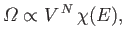(6.6)

where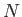is the number of atoms, and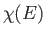depends only on the energy of the gas (and is, therefore, independent of the volume). We obtained this result by integrating over the volume of accessible phase-space. Because the energy of an ideal gas is independent of the atomic coordinates (given that there are no interatomic forces in an ideal gas), the integrals over these coordinates just reduced tosimultaneous volume integrals, giving the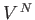factor in the previous expression. The integrals over the particle momenta were more complicated, but were clearly independent of, giving thefactor in the previous expression. Consider the following statistical result [see Equation (5.41)]: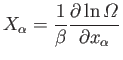(6.7)

where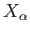is the mean force conjugate to the external parameter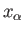(i.e.,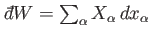), and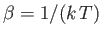. For an ideal gas, the only external parameter is the volume, and its conjugate force is the pressure (because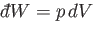). So, we can write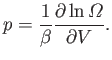(6.8)

It immediately follows from Equation (6.6) that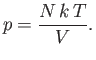(6.9)

However,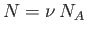, whereis the number of moles, and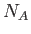is Avagadro's number. Also,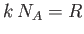, where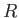is the molar ideal gas constant. This allows us to write the equation of state in its standard form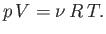(6.10)

Incidentally, the fact that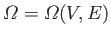in Equation (6.6) suggests that the macroscopic state of an ideal gas can be uniquely specified by giving the values of two independent parameters (e.g., the energy and the volume, the pressure and the volume, the temperature and the volume, et cetera).

The previous derivation of the ideal gas equation of state is rather elegant. It is certainly far easier to obtain the equation of state in this manner than to treat the atoms that make up the gas as tiny billiard balls continually bouncing off the walls of a container. The latter derivation is difficult to perform correctly, because it is necessary to average over all possible directions of atomic motion. (See Section 7.16.) It is clear, from the previous derivation, that the crucial element needed to obtain the ideal gas equation of state is the absence of interatomic forces. This automatically gives rise to a variation of the number of accessible states with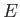andof the form (6.6), which, in turn, implies the ideal gas law. So, the ideal gas law should also apply to polyatomic gases with no inter-molecular forces. Polyatomic gases are more complicated that monatomic gases because the molecules can rotate and vibrate, giving rise to extra degrees of freedom, in addition to the translational degrees of freedom of a monatomic gas. In other words,, in Equation (6.6), becomes much more complicated in polyatomic gases. However, as long as there are no inter-molecular forces, the volume dependence of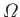is still, and the ideal gas law should still hold true. In fact, we shall discover that the extra degrees of freedom of polyatomic gases manifest themselves by increasing the specific heat capacity. (See Section 7.13.)

There is one other conclusion that we can draw from Equation (6.6). The statistical definition of temperature is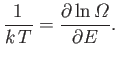(6.11)

[See Equation (5.31).] It follows that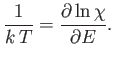(6.12)

We can see that, because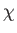is a function of the energy, but not of the volume, the temperature must also be a function of the energy, but not the volume. This implies that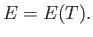(6.13)

In other words, the internal energy of an ideal gas depends only on the temperature of the gas, and is independent of the volume. This is a fairly obvious result, because if there are no inter-molecular forces then increasing the volume, which effectively increases the mean separation between molecules, is not going to affect the molecular energies. Hence, the energy of the whole gas is unaffected.

The volume independence of the internal energy can also be derived directly from the ideal gas equation of state. The internal energy of a gas can be considered to be a general function of the temperature and volume, so that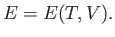(6.14)

It follows from mathematics that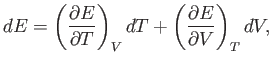(6.15)

where the subscriptreminds us that the first partial derivative is taken at constant volume, and the subscriptreminds us that the second partial derivative is taken at constant temperature. The first and second laws of thermodynamics imply that for a quasi-static change of parameters,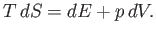(6.16)

[See Equation (6.5).] The ideal gas equation of state, (6.10), can be used to express the pressure in term of the volume and the temperature in the previous expression: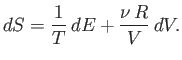(6.17)

Using Equation (6.15), this becomes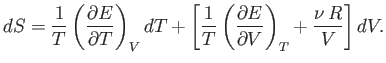(6.18)

However,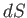is the exact differential of a well-defined state function,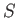. This means that we can consider the entropy to be a function of the temperature and volume. Thus,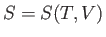, and mathematics immediately yields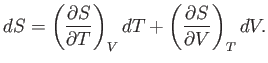(6.19)

The previous expression is valid for all small values of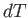and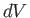, so a comparison with Equation (6.18) gives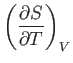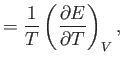(6.20)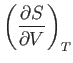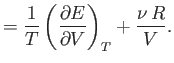(6.21)

A well-known property of partial differentials is the equality of second derivatives, irrespective of the order of differentiation, so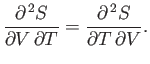(6.22)

This implies that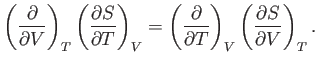(6.23)

The previous expression can be combined with Equations (6.20) and (6.21) to give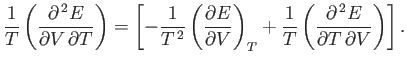(6.24)

Because second derivatives are equivalent, irrespective of the order of differentiation, the previous relation reduces to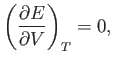(6.25)

which implies that the internal energy is independent of the volume for any gas obeying the ideal equation of state. This result was confirmed experimentally by James Joule in the middle of the nineteenth century.Next: Specific Heat Up: Classical Thermodynamics Previous: Introduction
Richard Fitzpatrick 2016-01-25